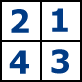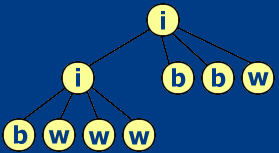시간 제한메모리 제한제출정답맞힌 사람정답 비율
1 초 128 MB20000.000%

## 문제

A binary image is an image containing pixels colored by only two colors, either black or white. We assume that each binary image is composed by N x N pixels, where N is a power of 2 and N<=1024. Given two binary images I1 and I2, their intersection is a new binary image I3, such that a pixel in I3 is black if and only if it is black in both I1 and I2.

A quadtree is a rooted tree which represents a binary image. The root corresponds to the whole image. If the image is one colored (black or white), the quadtree contains only the root labeled with 'b' if ot is black, and 'w' if it is white. Otherwise, the root is labeled 'i' (standing for "internal"), and the image is split to 4 equal parts (see figure), which are also binary images. For each of these new subimages, the procedure is the same. If any of these 4 subimages is 0-colored or 1-colored, then it is labeled 'b' or 'w' respectively. Otherwise, it is split in 4 sub-subimages.

This procedure may be repeated until the pixel level at most.

The numbering of the children of a parent node is illustrated in the following figure. Subquadrant 1 is placed as the left-most child, and subquadrant 4 is placed as the right-most child in the quadtree representation. The same rule is followed recursively for all the levels of the quadtree.Image 1 Image 2 Image 3

In the pre-order traversal of a quadtree, we first visit the root. Then, after the root we visit its 4 children (if they exist), following the above given ordering. For each of these 4 children, the same rule applies in a recursive way, where every child is taken as the root of the corresponding subtree.

Your job is, given the preorder traversal of two quadtrees (corresponding to two images) to find the number of nodes contained in the quadtree of their intersection.

## 입력

Your program should read the input from standard input as follows. The first line contains a positive integer number denoting the size N of the images. The next two lines contain the preorder strings of the quadtrees of two images (one string per line). Each string represents the preorder traversal of the quadtree of the corresponding image. In the preorder string, three characters are allowed: 'i' denoting an internal node, 'b' denoting a black quadrant and 'w' denoting a white quadrant.

## 출력

Your program should write the output into standard output. The file containes one line, denoting the total number of nodes (leaf + internal) of the quadtree corresponding to the intersection.

## 예제 입력 1

4
iiwwwbibbwwbw
iwbwiwwbw


## 예제 출력 1

9